# Let's roll dice!

{tidydice} 1.0.0 is here!

## Why {tidydice}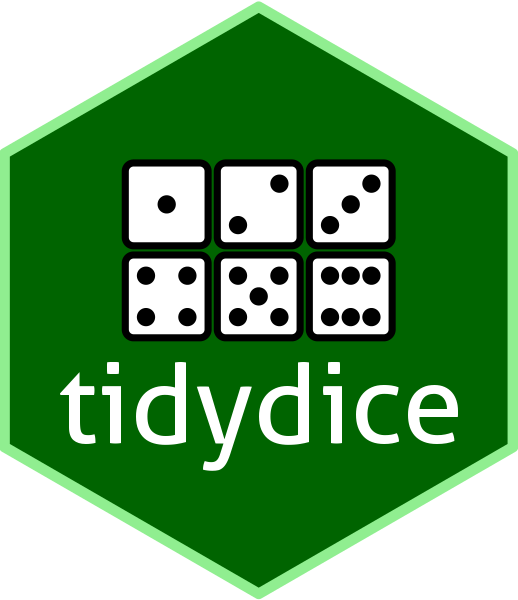A basic understanding of probability and statistics is crucial for data understanding. A great way to teach probability and statistics is to start with an experiment, like rolling a dice or flipping a coin.

{tidydice} simulates rolling a dice and flipping a coin. Each experiment generates tidy data (tibble). Dice rolls and coin flips are simulated using sample(). The properties of the dice can be changed, like the number of sides. A coin flip is simulated using a two sided dice. Experiments can be combined with the pipe-operator.

## Design

The default dice design is black/white with gold as highlight for a success. To make it reproducible, use `seed`

``````# use package
library(tidydice)

# example: roll dice 6 times
roll_dice(times = 6, seed = 123) |>
plot_dice()
``````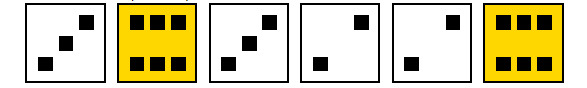You can control the design of dice using `plot_dice()` arguments:

• `fill` = fill color of dice
• `fill_success` = fill color of dice if result is a success
• `point_color` = color of points
• `line_color` = color of lines
• `line_size` = size of lines
``````roll_dice(times = 6, seed = 123) |>
plot_dice(fill = "darkgrey",
fill_success = "darkblue",
line_color = "white",
point_color = "white")
``````## Repeat

We see 2 six. This is more than th expected value, which is 1. (A dice has 6 sides, so the probability of getting a six is 1/6)

Now let’s repeat this experiment 6 times

``````roll_dice(times = 6, rounds = 6, seed = 123) |>
plot_dice(fill = "darkgrey",
fill_success = "darkblue",
line_color = "white",
point_color = "white")
``````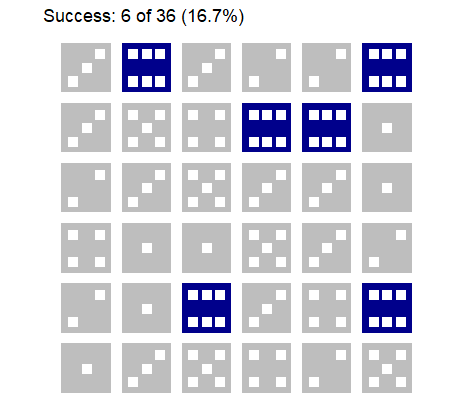Now we get 6 six. This is excactly the expected value.

## Aggregate

Lets increase rounds to 10 000. To see how many times we got a six in each round, use `agg = TRUE`

``````roll_dice(times = 6, rounds = 10000, agg = TRUE, seed = 123)
``````
``````# A tibble: 10,000 × 4
experiment round times success
<int> <int> <int>   <int>
1          1     1     6       2
2          1     2     6       2
3          1     3     6       0
4          1     4     6       0
5          1     5     6       2
6          1     6     6       0
7          1     7     6       0
8          1     8     6       1
9          1     9     6       0
10          1    10     6       1
# … with 9,990 more rows
``````

We get a tibble, where each row represents one round.

Let’s use {explore} to visualise the result

``````roll_dice(times = 6, rounds = 10000, agg = TRUE, seed = 123) |>
explore(success)
``````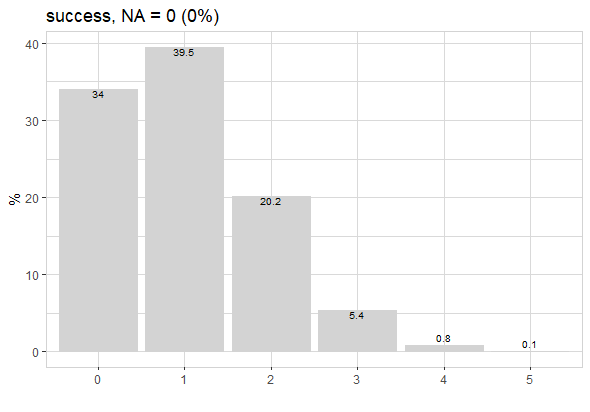## Binomial Distribution

By repeating the experiment 10 000 times we already get pretty close to the Binomial Distribution:

``````binom_dice(times = 6)
``````
``````# A tibble: 7 × 3
success         p      pct
<int>     <dbl>    <dbl>
1       0 0.335     33.5
2       1 0.402     40.2
3       2 0.201     20.1
4       3 0.0536     5.36
5       4 0.00804    0.804
6       5 0.000643   0.0643
7       6 0.0000214  0.00214
``````
``````binom_dice(times = 6) |>
plot_binom(highlight = 3:6)
``````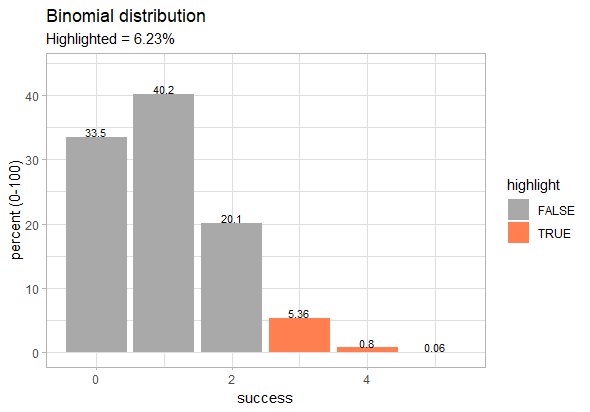The chance to get 3 or more six is just 6.23%

## Cheating

We can cheat by increasing the probability of getting a six from 1/6 (0.167) to 1/2 (0.5).

``````roll_dice(
times = 6,
prob = c(0.1,0.1,0.1,0.1,0.1,0.5),
seed = 123
) |> plot_dice()
``````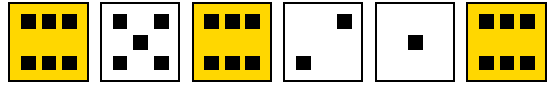Can this cheating be detected? 3 six is still in a normal range.

Let’s do an experiment!

``````roll_dice(times = 6, rounds = 10000, agg = TRUE) |>
roll_dice(
times = 6, rounds = 10000, agg = TRUE,
prob = c(0.1,0.1,0.1,0.1,0.1,0.5)) |>
explore(success, target = experiment)
``````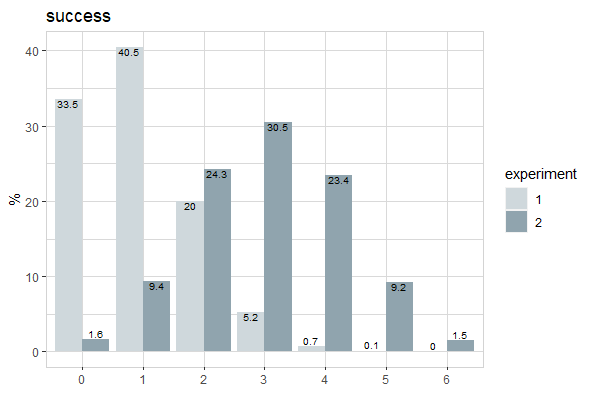The plot shows, that there is a high chance that the cheating will be detected, if we roll the dice multiple times. Because getting 4 or more six is very unlikely if there is no cheating.

### Using Dice Formula

If you want to do more complex dice rolls, use `roll_dice_formula()` (many thanks to Giovanni Marco for contributing the code!)

``````roll_dice_formula(
dice_formula = "4d6", # 4 dice with 6 sides
success = 15:24,      # success: sum between 15 and 24
seed = 123            # random seed to make it reproducible
)
``````
``````# A tibble: 1 × 7
experiment dice_formula label round    nr result success
<int> <chr>        <chr> <int> <int>  <dbl> <lgl>
1          1 4d6          4d6       1     1     18 TRUE
``````
``````# 4 dice with 6 sides + 1 dice with 10 sides
# repeat 1000 times
roll_dice_formula(
dice_formula = "4d6+1d10",
rounds = 1000) |>
explore_bar(result, numeric = TRUE)
``````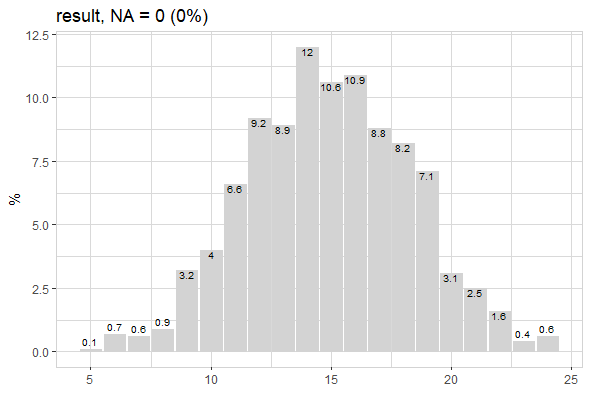Other examples for dice_formula:

• `1d6` = roll one 6-sided dice
• `1d8` = roll one 8-sided dice
• `1d12` = roll one 12-sided dice
• `2d6` = roll two 6-sided dice
• `1d6e6` = roll one 6-sided dice, explode dice on a 6
• `3d6kh2` = roll three 6-sided dice, keep highest 2 rolls
• `3d6kl2` = roll three 6-sided dice, keep lowest 2 rolls
• `4d6kh3e6` = roll four 6-sided dice, keep highest 3 rolls, but explode on a 6
• `1d20+4` = roll one 20-sided dice, and add 4
• `1d4+1d6` = roll one 4-sided dice and one 6-sided dice, and sum the results
Written on February 2, 2023